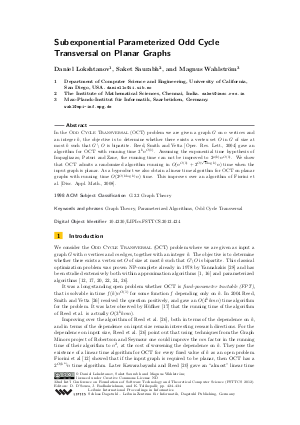Document# Subexponential Parameterized Odd Cycle Transversal on Planar Graphs

### Authors Daniel Lokshtanov, Saket Saurabh, Magnus Wahlström## File

LIPIcs.FSTTCS.2012.424.pdf
• Filesize: 0.51 MB
• 11 pages

## Cite As

Daniel Lokshtanov, Saket Saurabh, and Magnus Wahlström. Subexponential Parameterized Odd Cycle Transversal on Planar Graphs. In IARCS Annual Conference on Foundations of Software Technology and Theoretical Computer Science (FSTTCS 2012). Leibniz International Proceedings in Informatics (LIPIcs), Volume 18, pp. 424-434, Schloss Dagstuhl - Leibniz-Zentrum für Informatik (2012)
https://doi.org/10.4230/LIPIcs.FSTTCS.2012.424

## Abstract

In the Odd Cycle Transversal (OCT) problem we are given a graph G on n vertices and an integer k, the objective is to determine whether there exists a vertex set O in G of size at most k such that G - O is bipartite. Reed, Smith and Vetta [Oper. Res. Lett., 2004] gave an algorithm for OCT with running time 3^kn^{O(1)}. Assuming the exponential time hypothesis of Impagliazzo, Paturi and Zane, the running time can not be improved to 2^{o(k)}n^{O(1)}. We show that OCT admits a randomized algorithm running in O(n^{O(1)} + 2^{O(sqrt{k} log k)}n) time when the input graph is planar. As a byproduct we also obtain a linear time algorithm for OCT on planar graphs with running time O(n^O(1) + 2O( sqrt(k) log k) n) time. This improves over an algorithm of Fiorini et al. [Disc. Appl. Math., 2008].
##### Keywords
• Graph Theory
• Parameterized Algorithms
• Odd Cycle Transversal

## Metrics

• Access Statistics
• Total Accesses (updated on a weekly basis)
0